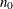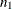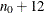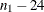### ESTIMATE Statement

`ESTIMATE` <options> ;

The ESTIMATE statement is an optional statement used to control the overall model-fitting environment. Using this statement, you can control the span of observations used to fit the model by using the SKIPFIRST= and BACK= options. This can be useful in model diagnostics. You can request a variety of goodness-of-fit statistics and other model diagnostic information including different residual diagnostic plots. Note that the ESTIMATE statement is not used to control the nonlinear optimization process itself. That is done using the NLOPTIONS statement, where you can control the number of iterations, choose between the different optimization techniques, and so on. You can save the estimated parameters and other related information in a data set by using the OUTEST= option. You can request the optimization of the profile likelihood, the likelihood obtained by concentrating out a disturbance variance, for parameter estimation by using the PROFILE option. The following example illustrates the use of this statement:

```   estimate skipfirst=12 back=24;
```

This statement requests that the initial 12 measurements and the last 24 measurements be excluded during the model-fitting process. The actual observation span used to fit the model is decided as follows: Suppose thatandare the observation numbers of the first and the last nonmissing values of the response variable, respectively. As a result of SKIPFIRST=12 and BACK=24, the measurements between observation numbersandform the estimation span. Of course, the model fitting might not take place if there are insufficient data in the resulting span. The model fitting does not take place if there are regressors in the model that have missing values in the estimation span.

BACK=integer
SKIPLAST=integer

indicates that some ending part of the data needs to be ignored during the parameter estimation. This can be useful when you want to study the forecasting performance of a model on the observed data. BACK=10 results in skipping the last 10 measurements of the response series during the parameter estimation. The default is BACK=0.

enables continuation of the diffuse filtering iterations foradditional iterations beyond the first instance where the initialization of the diffuse state would have otherwise taken place. If the specifiedis larger than the sample size, the diffuse iterations continue until the end of the sample. Note that one-step-ahead residuals are produced only after the diffuse state is initialized. Delaying the initialization leads to a reduction in the number of one-step-ahead residuals available for computing the residual diagnostic measures. This option is useful when you want to ignore the first few one-step-ahead residuals that often have large variance.

NOPROFILE

requests that the usual likelihood be optimized for parameter estimation. For more information, see the section Parameter Estimation by Profile Likelihood Optimization.

OUTEST=SAS-data-set

specifies an output data set for the estimated parameters.

In the ESTIMATE statement, the PLOT= option is used to obtain different residual diagnostic plots. The different possibilities are as follows:

PLOT=ACF
PLOT=MODEL
PLOT=LOESS
PLOT=HISTOGRAM
PLOT=PACF
PLOT=PANEL
PLOT=QQ
PLOT=RESIDUAL
PLOT=WN
PLOT=( <plot request> …<plot request> )

requests different residual diagnostic plots. The different options are as follows:

ACF

produces the residual-autocorrelation plot.

CUSUM

produces the plot of cumulative residuals against time.

CUSUMSQ

produces the plot of cumulative squared residuals against time.

MODEL

produces the plot of one-step-ahead forecasts in the estimation span.

HISTOGRAM

produces the histogram of residuals.

LOESS

produces a scatter plot of residuals against time, which has an overlaid loess-fit.

PACF

produces the residual-partial-autocorrelation plot.

PANEL

produces a summary panel of the residual diagnostics consisting of

• histogram of residuals

• normal quantile plot of residuals

• the residual-autocorrelation-plot

• the residual-partial-autocorrelation-plot

QQ

produces a normal quantile plot of residuals.

RESIDUAL

produces a needle plot of residuals against time.

WN

produces a plot of p-values, in log-scale, at different lags for the Ljung-Box portmanteau white noise test statistics.

PRINT=NONE

suppresses all the printed output related to the model fitting, such as the parameter estimates, the goodness-of-fit statistics, and so on.

PROFILE

requests that the profile likelihood, obtained by concentrating out one of the disturbance variances from the likelihood, be optimized for parameter estimation. By default, the profile likelihood is not optimized if any of the disturbance variance parameters is held fixed to a nonzero value. For more information see the section Parameter Estimation by Profile Likelihood Optimization.

SKIPFIRST=integer

indicates that some early part of the data needs to be ignored during the parameter estimation. This can be useful if there is a reason to believe that the model being estimated is not appropriate for this portion of the data. SKIPFIRST=10 results in skipping the first 10 measurements of the response series during the parameter estimation. The default is SKIPFIRST=0.